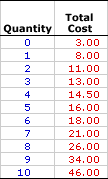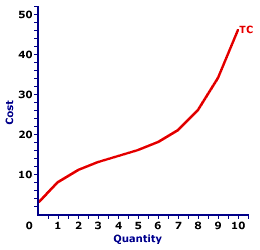Saturday  September 23, 2023
 AmosWEB means Economics with a Touch of Whimsy!MARGINAL REVENUE CURVE, MONOPOLISTIC COMPETITION: A curve that graphically represents the relation between marginal revenue received by a monopolistically competitive firm for selling its output and the quantity of output sold. The marginal revenue curve reflects the degree of market control held by a firm. For a monopolistically competitive firm with some market control, but not a whole lot, the marginal revenue curve is negatively-sloped but relatively elastic.TOTAL COST:

The opportunity cost incurred by all of the factors of production used by a firm to produce a good or service, including wages paid to labor, rent paid for the land, interest paid to capital owners, and a normal profit paid to entrepreneurs. Total cost is most important in the analysis a firm's short-run production decision and is frequently separated into total variable cost and total fixed cost. Two other cost measures directly related to total cost are marginal cost and average total cost. Total cost is half of the information a firm uses to determine profit, the other half is total revenue.
Total cost is the overall opportunity cost incurred by a firm in production. For short-run production, total cost consists of variable cost, which depends on the quantity produced, and fixed cost, which does not vary with production. The variable component of total cost, is guided by the law of diminishing marginal returns and is key to short-run production and supply decisions.

Consider the cost incurred by The Wacky Willy Company in the production of Wacky Willy Stuffed Amigos (a complete line of adorable, huggable, collectable stuffed animals). The production of Stuffed Amigos requires materials from the land (fuzzy cloth, soft stuffing, thread), labor to do the fabrication and assembly (cloth cutters, seam sewers, stuffing stuffers), and capital equipment to make the workers more efficient (sewing machines, scissors). Of course, Stuffed Amigos production also requires entrepreneurs to organize and finance the whole process.

Each of these resources incur an opportunity cost to produce Stuffed Amigos. Each could have been used to produce other goods. Wages compensate workers who could have been producing Hot Momma Fudge Bananarama Ice Cream Sundaes. The material suppliers could have supplied their materials for the production of OmniHome pillows and sheets. The capital and equipment could have been used in the production of the Prancing Pistachio line of pet clothing. And the organizers, the entrepreneurs, could have organized the production of Flex-Star Interactive Trophy Plaques, Auntie Noodles Frozen Macaroni Dinner, or Double-Dot Carmel Nougat Clusters. Total cost includes the compensation to all of these resources for NOT doing other production.

### Total Stuffed Animal Cost

Total CostThe table to the right provides hypothetical cost numbers incurred by The Wacky Willy Company in the production of Stuffed Amigos. These numbers can provide a little insight into total cost.

The column to the left presents the quantity of Stuffed Amigos produced each minute, ranging from 0 to 10 stuffed animals. The column to the right then presents the total cost incurred in this production, ranging from a low of \$3 to a high of \$46. If, for example, 5 Stuffed Amigos rolls off the assembly line per minute, then the total cost incurred in their production is \$16. The production of 9 Stuffed Amigos, in comparison, incurs a total cost of \$34.

Closer consideration of these total cost numbers is in order.

• Short-run Production: This table of numbers is for the short-run production of Stuffed Amigos, which means at least one input is fixed and at least one input is variable. The fixed input is the Wacky Willy factory and associated capital equipment, and the variable inputs are workers and materials.

• Increasing Total Cost: The most obvious point, as such, is that total cost increases with increased production. Producing more Stuffed Amigos means higher total cost. This makes sense. To produce more Stuffed Amigos, The Wacky Willy Company needs to hire more labor and buy more materials. Because these inputs are not free, total cost rises with extra production.

• Fixed Cost: However, total cost is not zero when no Stuffed Amigos are produced. Curious, eh? The Wacky Willy Company incurs \$3 of cost even if they employ no workers or buy no materials--their variable inputs. The reason is that they must continue to pay the costs of any fixed inputs, especially capital. This \$3 is the total fixed cost incurred in the short-run production of Stuffed Amigos. It is paid whether or not any Stuffed Amigos are produced.

• Marginal Cost: A last point to note in passing is that the incremental increase in total cost is NOT the same for each quantity. In other words, total cost does not rise at a constant rate. This is reflected by the notion of marginal cost, which plays a pivotal role in the short-run production analysis.

### Fixed and Variable

The total cost incurred in the short-run production of goods like Wacky Willy Stuffed Amigos can be divided into two components--fixed and variable.
• Fixed Cost: This is the opportunity cost of production that does NOT vary with the quantity of output produced. In other words, the same level of fixed cost is incurred regardless of the quantity produced. Most, not all but most, fixed cost is associated with the use of fixed inputs in the short run.

The Wacky Willy Company, for example, incurs a fixed level of "overhead expenses" that include administrative salaries, interest on the loan used to buy capital equipment, and rent on the building. Whether The Wacky Willy Company produces one Stuffed Amigo or one million Stuffed Amigos, this fixed cost does not change. The table above indicates that The Wacky Willy Company incurs \$3 of fixed cost each minute of production, which is the cost incurred if production is zero.

• Variable Cost: This is the opportunity cost of production that does vary with the quantity of output produced. In other words, if production changes, then variable cost changes, too. Most, not all but most, variable cost is based on the use of variable inputs.

The Wacky Willy Company, for example, incurs variable cost when it hires a different number of workers or buys a different quantity of material inputs, both of which are done as it changes the quantity of output produced. That is, if The Wacky Willy Company wants to produce more Stuffed Amigos, then it must buy more materials and hire more workers. The table above indicates any cost greater than \$3 per minute incurred by The Wacky Willy Company is variable cost.

The division of total cost between total fixed cost and total variable cost is often summarized in this handy equation:
 total cost = total fixed cost + total variable cost

### The Total Cost Curve

Total Cost CurveLike most relations found in the study of economics, that between total cost and the quantity of production can be represented by a curve. The total cost curve is presented in the exhibit to the right.

As might be expected the total cost curve has a positive slope. As the quantity of output produced increases, so too does total cost. However, the slope of the total cost curve is not constant. It is relatively steep for small quantities, flattens for intermediate levels of production, then once again steepens for the largest quantities. The shape of the total cost curve is based on short-run production returns, especially the law of diminishing marginal returns.

Another observation is that the total cost curve does not go through the origin, but rather begins at a positive value on the vertical axis. In other words, if the quantity of output is zero, total cost is positive. This vertical intercept indicates fixed cost.

### Short-run Production Returns

The shape of the total cost curve reflects short-run production returns. In the short run, with at least one fixed input and at least one variable input, production is guided by increasing marginal returns for small quantities of output, then decreasing marginal returns for larger quantities.
• Increasing Marginal Returns: Increasing marginal returns are reflected by the flattening slope for small quantities. With increasing marginal returns, fewer variable inputs are needed to produce a given incremental increase in output. As such, total cost increases, but at a lessening pace.

• Decreasing Marginal Returns: In contrast, decreasing marginal returns are reflected by the increasing slope for larger quantities. With decreasing marginal returns, more variable inputs are needed to produce a given incremental increase in output. As such, total cost increases at an increasing rate.
Most important to these observations is the role played by the law of diminishing marginal returns. Decreasing returns incurred for larger quantities of output are caused by the law of diminishing marginal returns. As such, total cost increases at an increasing pace, and the total cost curve becomes increasingly steeper, due to the law of diminishing marginal returns.

### Average and Marginal

Total cost provides the foundation for short-run production analysis. Two key cost concepts derived from total cost are average cost and marginal cost.
• Average Cost: In general, average cost is simply the cost per unit of output. It is the total cost divided by the quantity of output produced. If, for example, the total cost of producing 10 Stuffed Amigos is \$46, then the average cost is \$4.60 (= \$46/10). A specific average derived from total cost is termed average total cost.

• Marginal Cost: A second cost concept is the change in total cost resulting from a change in quantity of output. Marginal cost is specified as the change in total cost divided by the change in the quantity of output produced. If, for example, the total cost is \$34 for producing 9 Stuffed Amigos and \$46 for producing 10 Stuffed Amigos, then the marginal cost is \$12. Total cost increases by \$12 with the production of one additional Stuffed Amigos.
While average cost (especially average total cost) plays a key role in the analysis of short-run production, marginal cost is far and away more important. Marginal cost guides the short-run production decision of a firm as it selects the profit maximizing quantity of output to supply.

 <= TIME DEPOSITS TOTAL COST AND MARGINAL COST =>Recommended Citation:

TOTAL COST, AmosWEB Encyclonomic WEB*pedia, http://www.AmosWEB.com, AmosWEB LLC, 2000-2023. [Accessed: September 23, 2023].

Check Out These Related Terms...

Or For A Little Background...

And For Further Study...
Search Again?GRAY SKITTERY[What's This?] Today, you are likely to spend a great deal of time looking for a downtown retail store looking to buy either a T-shirt commemorating the 2000 Presidential election or a really, really exciting, action-filled video game. Be on the lookout for pencil sharpeners with an attitude.Your Complete ScopeRagnar Frisch and Jan Tinbergen were the 1st Nobel Prize winners in Economics in 1969."People of mediocre ability sometimes achieve outstanding success because they don't know when to quit. "-- George Allen, U.S. senatorIERInternational Economic ReviewA PEDestrian's Guide Xtra CreditTell us what you think about AmosWEB. Like what you see? Have suggestions for improvements? Let us know. Click the User Feedback link.| | | | | | | | | | |
| | | |

Thanks for visiting AmosWEB